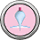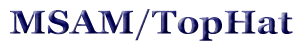## Balloon Experiment: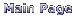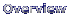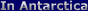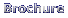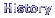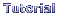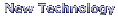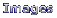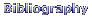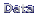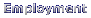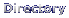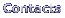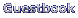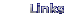For my experiment, TopHat, we have a certain amount of instruments that have to go on the balloon, some on top, some on bottom. However, the more stuff we add to the balloon, the bigger the balloon needs to be. There is certainly a limit to how big helium balloons can get, so there must be a limit to how many instruments it can carry. Also, there are some issues of balance that need to be dealt with. Our objective in this lab is to get a sense of some of the limitations and problems scientists must deal with in order to accomplish what they were trying to do.

Pre-experiment questions

*For your reference, a medium sized paper clip weighs about 1 gram.*

1. Just by guessing, how many grams do you think a balloon can carry before it sinks to the ground?

2. How much weight do you think is possible to balance on top of the balloon before it tips over?

3. Is it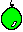possible to make the bottom part of a balloon (the tied part) become the top part and still float? If yes, guess a way you could do this. If no, explain why not.

Materials needed

• 11" helium balloons, enough for each pair of students to get one
• lots of small paperclips, each pair should need around 30 clips
• some scotch tape (to attach the paperclips to the top of the balloon)
• a gram scale
• an ordinary bathroom scale

Procedure

 Point 1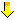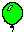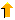Point 2
The class should divide itself into groups of 2. Each pair should receive a balloon and several paperclips. To start out with, each team should find the topmost point on the balloon and indicate it with a marker. I will refer to this spot as point 1 from now on. The spot where the balloon was tied closed will be referred to as point 2.

Part I:

Step 1- What is the total amount of weight your balloon can hold without sinking to the ground? Record this amount. Remove the weight from the balloon.

Step 2- What is the maximum amount of weight that can be attached to point 1 and still have it be the top of the balloon? Record this amount.

Step 3- How much weight can now be attached to point 2 (with the maximum weight from Step 2 still on point 1) without the balloon sinking to the floor? Record your answer.

Step 4- Add your measurements from Step 2 and Step 3. Is this the same number as you got for Step 1? Should it be?

Step 5- Take the data from Step 2 and divide it by the number from Step 4. Then multiply this number by 100. This number is the PERCENT of the total weight the balloon can carry (your answer from Step 1) that can be balanced when point 1 is the top of the balloon. Surprising? Remove the weights.

Part II:

Step 6- What is the minimum amount of weight that has to be attached to point 1 in order to make it be the bottom of the balloon? Record this.

Step 7- How much weight can be attached to point 2 (with the minimum weight of Step 6 still on point 1) without the balloon sinking to the floor? Record.

Step 8- Take your answer from Step 7 and divide it be the number from Step 1. Then multiply this number by 100. This number is the percent of total weight the balloon can carry when point 2 is the top of the balloon. Why are the numbers from Step 5 and Step 8 different?

Step 9- What is your weight? You probably know your weight in pounds, but all our earlier measurements were in grams, so we need to convert your weight to grams. To do that, multiply your weight by 453.6. Now divide your weight in grams by the number from Step 1. This is the amount of balloons it would take to lift you into the air!

Step 10- In my experiment, TopHat, there is one big helium balloon. To give ourselves an idea of how big that balloon is, let's see how many of our little balloons it takes to be equal to one big balloon. The total weight of TopHat, in pounds, is 8300. Again, we need to convert this number to grams by multiplying by the number 453.6. Now, divide the weight of TopHat in grams by the answer from Step 1. Wow, that's a lot of balloons!

 [MAIN PAGE] [BROCHURE] [HISTORY] [TUTORIAL] [NEW TECHNOLOGY] [IMAGES] [BIBLIOGRAPHY] [DATA] [EMPLOYMENT] [DIRECTORY] [CONTACTS] [GUESTBOOK]